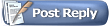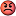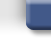Project64 ForumsBlog Space :D Sine of One Degree
 FAQ Members List Calendar Search Today's Posts Mark Forums Read#121HatCatAlpha Tester Project Supporter Senior Member Join Date: Feb 2007 Location: In my hat. Posts: 16,236Quote:
 Originally Posted by ExtremeDude2Bro, didn't I tell u that like 2 years ago?
Only thing was, it was my research project--your teacher's assignment.

I pretty much did all the work.Meanwhile your prof was asking you to do dumb C++ things to make the program even faster and deducted points from it or whatever.
#122ExtremeDude2Alpha Tester Project Supporter Senior Member Join Date: Apr 2010 Location: USA Posts: 3,118Bro, everyone knows I'm the best__________________
Quote:
 Originally Posted by dsx!are you american or something
#123HatCatAlpha Tester Project Supporter Senior Member Join Date: Feb 2007 Location: In my hat. Posts: 16,236To continue solving sin(1) any further from that stage seems to require messing around with DeMoivre's Theorem, in order to evaluate and rewrite cube roots of complex numbers. So I think I will put off that continuation for later.

So instead of immediately falling back to that, I've written my own solution for the 3-87-90 degree triangle (a right triangle with a base angle of 3 degrees), which solves sin(3), cos(3), tan(3) etc.:

Code:
```      B
r  /|
/  | y
/____|
A  x  C```
x: horizontal length of triangle ABC
y: vertical length of triangle ABC
r: vector resultant of segments AC and BC (alternatively the radius of circle A when B is one of the circle's points)Now sin(3) is something which has already been perfectly well documented and solved for in plenty of other projects and sites, but it is still difficult to simplify before using it as a base for other angles, like sin(1). So I wanted to test that on my own first.

Last edited by HatCat; 17th December 2015 at 09:57 PM.
#124HatCatAlpha Tester Project Supporter Senior Member Join Date: Feb 2007 Location: In my hat. Posts: 16,236Long time no update...

I've finished mostly a JavaScript port of an old project I began in 2007, over 8 years ago.
It solves any triangle for all 3 sides and all 3 angle measures, once enough info is supplied.

http://cxd4.github.io/trig

Does not need to be a right triangle...but if you input side lengths like (a, b, c) = (1, 2, 3) that is not a valid triangle so it spams the page with an error. Obtuse angles (theta > 90 degrees) sometimes make the WebGL render drawing span off the edge of the screen, not sure if that's even possible for me to fix.
#125HatCatAlpha Tester Project Supporter Senior Member Join Date: Feb 2007 Location: In my hat. Posts: 16,236New discovery!

I found that the nested complex radical expressions I denoted via a and b actually were alternative forms of expressing sin(3 degrees) and cos(3 degrees) ... a new image will help me do future continued analysis with this new detail in focus, although I can't guarantee it will make a difference. :/#126
 amybugbee8Junior Member Join Date: Jan 2016 Posts: 5It was just informative news sharing and it's really helpful for everyone.
#127HatCatAlpha Tester Project Supporter Senior Member Join Date: Feb 2007 Location: In my hat. Posts: 16,236Not purposely bumping this to make people mad.

Just been a few months and I neglected to post a new discovery I made.

If you solve the cubic:
Code:
```8*x*x*x - 6*x - 1 == 0
//8x^3 - 6x - 1 = 0```
We have the solutions:
Code:
```x0 = cos(pi/3/3 +   0*pi/180) - 0/3 = cos(pi/9)
x1 = cos(pi/3/3 +  60*pi/180) - 0/3 = cos(4/9 * pi)
x2 = cos(pi/3/3 + 120*pi/180) - 0/3 = cos(7/9 * pi)

x0 =  cos( 20 deg.) =  sin(70 deg.) ~= +0.93969262078590838405410927732473
x1 = -cos( 80 deg.) = -sin(10 deg.) ~= -0.17364817766693034885171662676931
x2 =  cos(140 deg.) = -cos(40 deg.) ~= -0.76604444311897803520239265055542```
Though, this cubic was only solvable with the trigonometric approach, due to the discriminant being negative.

As for how I discovered this cubic...

Quote:
 Originally Posted by amybugbee8It was just informative news sharing and it's really helpful for everyone.

Now it turns out that sin(1 deg.) is non-algebraic.
This means that the exact value of sin(pi / 180) can't be defined as an algebraic expression, short of taking complex roots of unreal numbers (in terms of sqrt(-1)).

So it turns out, it's impossible to find the exact value of sin(1 deg.) further than what I've already simplified it down to.

However, here's an interesting experiment I ran.
If you draw a convex regular nonagon (9 sides, all 140-degree interior angles):You can take the yellow-shaded triangle and set up proportions to similar triangles inside it:From this, the similarity of multiple triangles (and the laws of the equilateral triangle in the center) allow a cubic equation relationship of at least one side, giving the possibility of using that to get the exact value of cos(20 deg.) and, sequentially, cos(1 deg.) or the sin(1 deg.).

Cardano's work in the Renaissance to solve cubic equations proposed a "reduced cubic" formation, which the discovered cubic already conforms to.

Code:
```x^3 + ax + b = 0

8x^3 - 6x - 1 = 0
x^3 - .75x - .125 = 0
a = -0.75
b = -(1/8)```
The cubic equation discriminant:
Code:
```Q = a/3 = -3/4 * 1/3 = -1/4
R = -b/2 = 1/8 * 1/2 =  1/16
discriminant = Q^3 + R^2
discriminant = -3/256```
Um I need to make another post after I dig up my notes on how to plug that in though. I had the solution but my Notepad.exe got accidentally'd a few hours ago.
#128HatCatAlpha Tester Project Supporter Senior Member Join Date: Feb 2007 Location: In my hat. Posts: 16,236Right, now that I've found a computer with LibreOffice Math on it:The three "solutions" to the cubic are the 20-, 140- and 260-degree angles' co-sines. The 260-degree angle is really just taking the negative of cos(260 - 180 = 80 degrees), which is also -sin(90 - 80 = 10 degrees), and the 140-degree angle is just a 40-degree angle reflected across the y-axis.

With that all said, only the 20-degree angle was the one I could successfully find the cubic polynomial relation for. (See triangle dissection images in prior post.) The exact proof I had finished was a blend of sin, cos, tan and friends on the 20-degree angle: 8*sin(20 deg.)*cos^2(20 deg.) - 2*sin(20 deg.) = sqrt(3) = 4*sin(20 deg.) + tan(20 deg.), ending ultimately in: 8*cos^3(20 deg.) - 6*cos(20 deg.) - 1 = 0.

Now, dividing the problem [8x^3 - 6x - 1 = 0] by 8, immediately produces Cardano's reduced cubic form:Since the discriminant is negative, the problem of identifying the three real-number solutions (if disc. was positive, we'd have unreal-number solutions) as algebraic number expressions not in terms of i seems to become impossible.Only x0 is shown here, which is the most simplified of distractions from the problem of taking cube roots of unreal numbers. x1 and x2 are worth investigating too but not in this thread; it's time-consuming and only the 20-degree angle--not the others--was the source behind the ability to produce the original cubic to begin with.

Dividing by cuberoot(16) of course is not relevant to the root of the problem either.
Code:
```cos(20 deg.)           = (cbrt(1 + sqrt(-3)) + cbrt(1 - sqrt(-3))) / cbrt(16)
2*cos(20 deg.)         = (cbrt(1 + sqrt(-3)) + cbrt(1 - sqrt(-3))) / cbrt(2)
2*cbrt(2)*cos(20 deg.) = cbrt(1 + sqrt(-3)) + cbrt(1 - sqrt(-3))
4*cos(20 deg.)         = cbrt(4 + 4*sqrt(-3)) + cbrt(4 - 4*sqrt(-3))```

Last edited by HatCat; 6th October 2016 at 09:26 PM.
#129ExtremeDude2Alpha Tester Project Supporter Senior Member Join Date: Apr 2010 Location: USA Posts: 3,118Quote:
 Originally Posted by HatCatNot purposely bumping this to make people mad.
I am very triggered__________________
Quote:
 Originally Posted by dsx!are you american or somethingThread ToolsShow Printable VersionEmail this Page Display ModesLinear ModeSwitch to Hybrid ModeSwitch to Threaded ModePosting Rules You may not post new threads You may not post replies You may not post attachments You may not edit your posts BB code is On Smilies are On [IMG] code is On HTML code is Off
 Forum Jump User Control Panel Private Messages Subscriptions Who's Online Search Forums Forums Home General Discussion     Site News     Open Discussion Public Version     Project 64 - v2.x - Suggestions     Project 64 - v2.x - Issues     Project64 - Android     Project 64 - v1.6

All times are GMT. The time now is 05:34 PM.Contact Us - Archive - Top